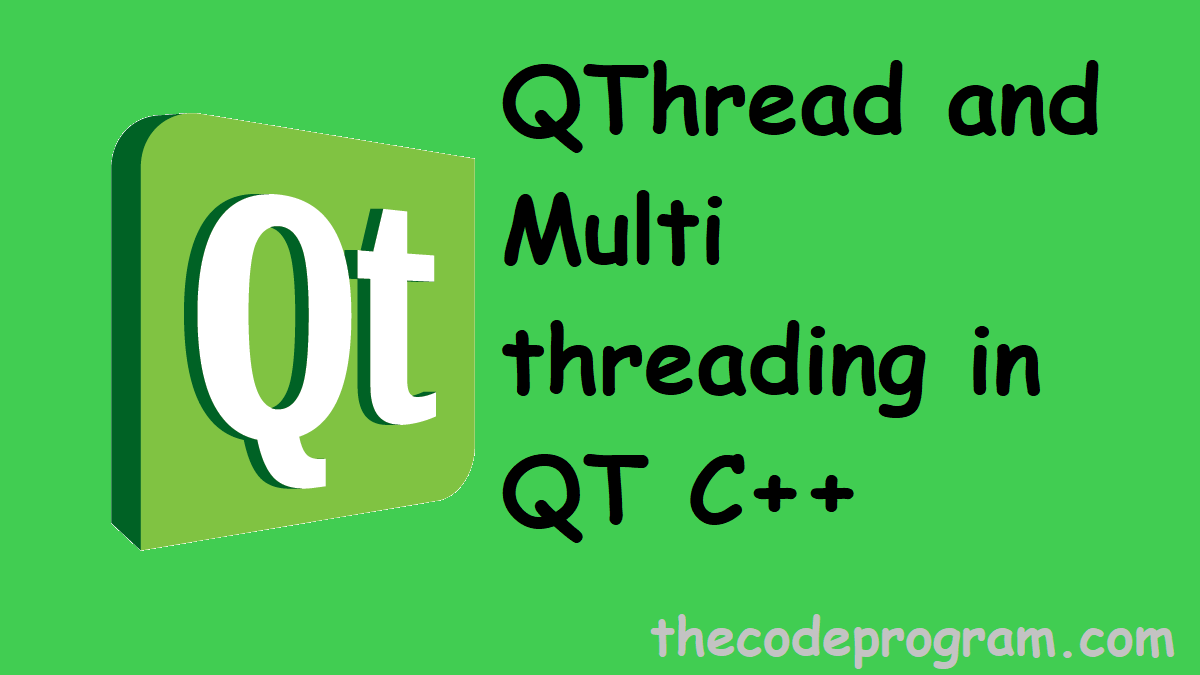Hello everyone in thiss article we are going to talk about usage of QThread class and implementation of Multithreading process in QT GUI C++ and we will make an example about it.

Now let's get started.

In this article I will create another class and I will define a Signal-Slot connection between my eanother C++ class and Mainwindow class. Ant then I will create a QThread which make callbacks to my class.

Now we need to create a signal slot connection. Below code block you can see my thread class header file content.
``````
``````
``````
{
Q_OBJECT

public:
explicit thread_calculate_value(QObject *parent = 0, bool b = false);
void run();

// if Stop = true, the thread will break
// out of the loop, and will be disposed
bool Stop;

signals:
// To communicate with Gui Thread
// we need to emit a signal
void have_calculatedVal(float ,    float  );

public slots:

};
``````

As you can see above code block, we have included the QThread library at the head of the class. After that the important thisn is implementation of QThread if we do not implement the QThread the class will not be thread class. And the class will not act as a thread class. Now we have implemented and our class will be act as a QThread class.

Another thing is here we have a signal named have_calculatedVal and it has two float typed variables. We will emit this signal from our thread C++ class. This emitting will transfer some datas to place which requested. Also this data transferring will not effect the main program thread and mainwindow GUI. We will do this almost one thousand times in a second and it will update the GUI and not freeze the GUI.

Now have a take a look at thread class:
``````

{
}

// run() will be called when this thread start
{
//to prevent consuming memory every time, I defined the variables at once
int calculated_a;
int calculated_b;

while(1)
{
emit have_calculatedVal(
calculated_a / 128.0,
calculated_b * 60.2
);
this->msleep(1);
}
}
``````

As you can see above code block will calculate some data and emit a signal with variables to the program. And also we are emiting the signal in a while loop and continuously. Just waiting for a second between every emiting. In normal program this action will freze the GUI and program will not be working efficiently.

Our thread is ready. Now we can run this thread from our mainwindow independently and let this thread to update our GUI without any freezing because of this calculations and while loop.

Now take a look at to our mainwindow header and C++ classes.

``````

class MainWindow : public QMainWindow
{
Q_OBJECT

public:
Ui::MainWindow *main_ui;

explicit MainWindow( QWidget *parent = 0 );
~MainWindow();

private:

public slots:
void set_calculatedValues(float airspeed,     float verticalSpeed );
};
``````
We have created the header file like above code block. Below code block you will see the C++ file.
``````
MainWindow::MainWindow( QWidget *parent ) :
QMainWindow( parent ),
main_ui( new Ui::MainWindow )
{
main_ui->setupUi( this );

connect( thr_calculate_value , SIGNAL(have_calculatedVal(float,float )),   this, SLOT(set_calculatedValues(float,float ))        );
thr_calculate_value->start();

}

void MainWindow::set_calculatedValues(float _cal_a,
float _cal_b)
{
lblCalculatedA->setText(_cal_a);
lblCalculatedB->setText(_cal_b);
}
``````

Now we are ready to use our thread. You can create threads how many thread do you need and you can start them as we talked about above.

Burak Hamdi TUFAN

04/12/2015

06/04/2020

30/08/2021